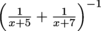# SAT Math: Using Common Denominators to Find an Equivalent Expression

Which of the following expressions is equivalent to?

 Passport to Advanced Math Solving quadratic equations Product Type SAT Math SAT SAT Math SAT Math Algebra and FunctionsPassport to Advanced Math Test Prep SAT Math

### Transcript

00:19

it The expression could just flip flop our minds completely

00:22

out of our skulls were not careful here So slow

00:24

down few breasts and find our way through it So

00:27

based on the answer choices it looks like this problem

00:29

wants us to turn this mess into one nice fractional

00:32

term Common denominators sound like a good starting point And

00:35

if you look at the answer choices here their way

00:37

00:40

starting with this mess right here and well then we're

00:43

going to smoosh the whole thing together simplify the numerator

00:46

and expand the denominator So that gets us quantity X

00:49

plus seven plus quantity x plus five over quantity Exposed

00:52

five times Explosive in all to the negative one And

00:56

well funny thing we have What now Looks like two

00:59

x plus twelve over X squared Plus twelve ax plus

01:03

thirty five Power Interesting What do you think You get

01:07

the negative one there That does not match It's wrong

01:10

It's not a so what the power of negative one

01:13

wants us to do remember is invert everything We invert

01:17

the fraction so it's actually going to look like this

01:19

X squared plus twelve expose thirty five over two X

01:22

plus twelve which is B which is the right answer